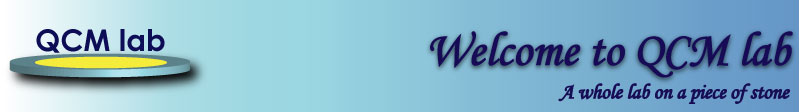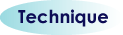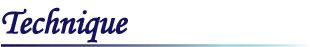Quartz Crystal Microbalance (QCM) technique uses the piezoelectric effect to develop very high accelerations on the surface of a quartz resonator vibrating in a shear mode. Thus, a mega-gravity inertial field is created on the surface of a quartz resonator. The recently formulated and demonstrated Acceleration Dependent Mass Sensitivity Principle explains the very high mass sensitivity of the QCM. It also explains the origins of frequency dependence on a liquid viscosity and helps the interpretation of the experimental results. QCM technique was introduced in 1959 by Sauerbrey. Since then it became a a largely used technique for mass measurements of the films adherently deposited on the surface of a quartz resonator. The resonant frequency of the quartz resonator changes when the the mass of the adherently deposited film changes. An increase in the film mass will induce a decrease of the resonant frequency. The mass change of a solid film can be accurately calculated using the equation derived from the Energy Transfer Model (ETM). This equation was experimentaly verified up to a mass load of 37%. The Sauerbrey's equation is accurate only for small mass loads under 2%. QCM-soft calculates the mass of the deposited film and its thickness using the equation derived from the ETM. The series resistance of a quartz resonator will increase when vibrating energy is dissipated in the contacting film or in the surrounding medium. Phase transitions or changes of the viscoelastic properties of the deposited film can be revealed by changes in the series resistance of the quartz resonator. The series resistance can also be used to make corrections in the mass calculations when viscoelastic films (proteins, polymers etc.) are deposited on the quartz resonator surface. This correction also depends on the crystal geometry and electrode design.